Скачать презентацию CHAPTER 7 Bonds and Their Valuation n n

348d0be65b13488418cd150dc02b917a.ppt

• Количество слайдов: 36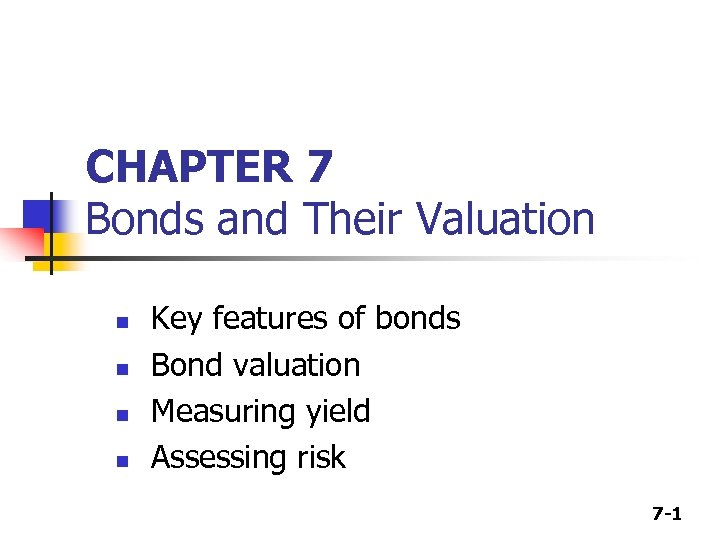CHAPTER 7 Bonds and Their Valuation n n Key features of bonds Bond valuation Measuring yield Assessing risk 7 -1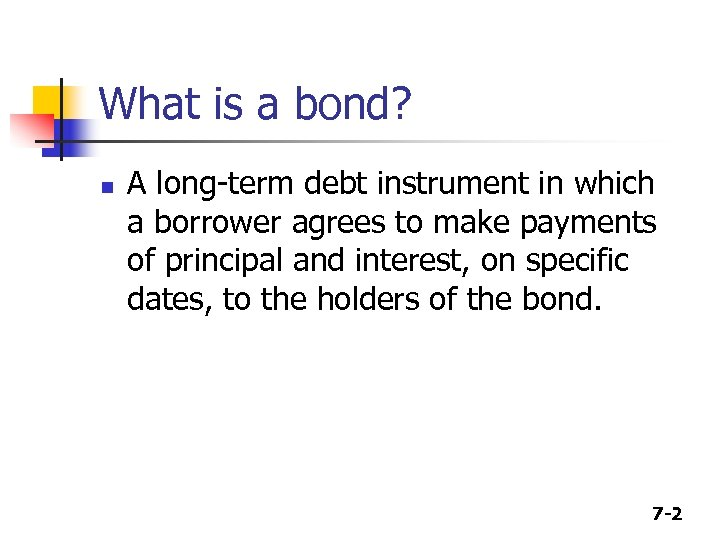What is a bond? n A long-term debt instrument in which a borrower agrees to make payments of principal and interest, on specific dates, to the holders of the bond. 7 -2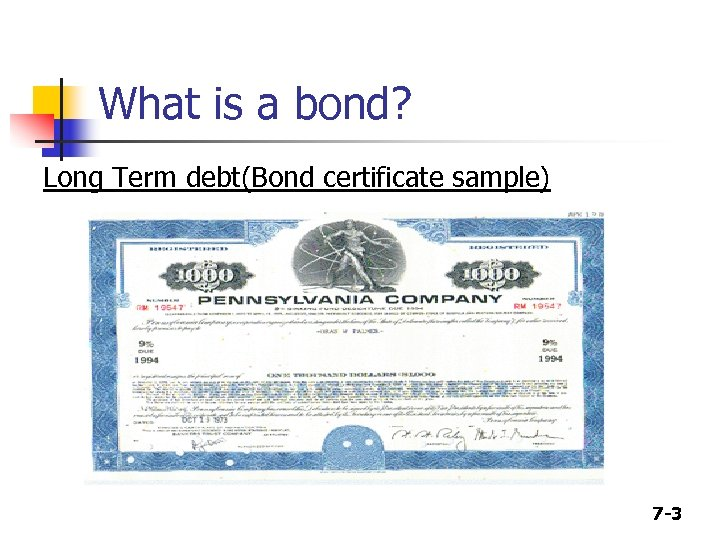What is a bond? Long Term debt(Bond certificate sample) 7 -3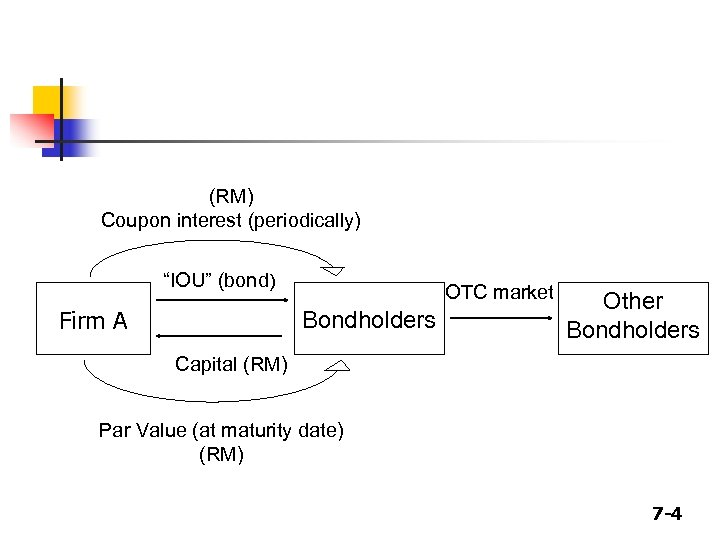(RM) Coupon interest (periodically) “IOU” (bond) Firm A OTC market Bondholders Other Bondholders Capital (RM) Par Value (at maturity date) (RM) 7 -4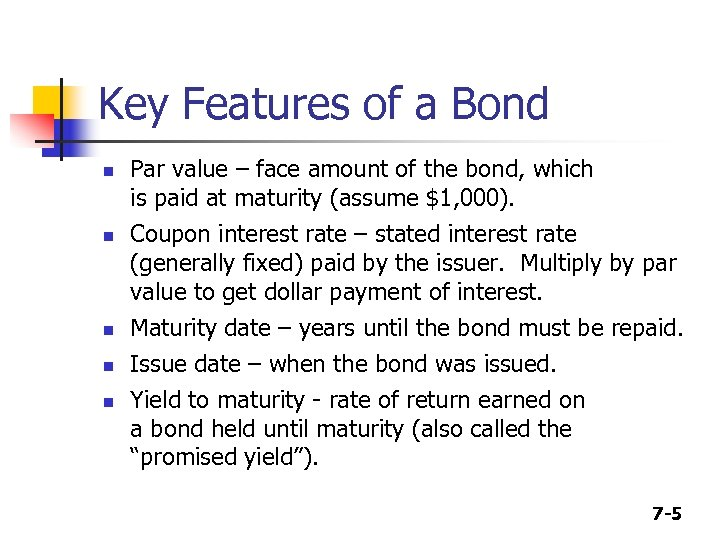Key Features of a Bond n n n Par value – face amount of the bond, which is paid at maturity (assume \$1, 000). Coupon interest rate – stated interest rate (generally fixed) paid by the issuer. Multiply by par value to get dollar payment of interest. Maturity date – years until the bond must be repaid. Issue date – when the bond was issued. Yield to maturity - rate of return earned on a bond held until maturity (also called the “promised yield”). 7 -5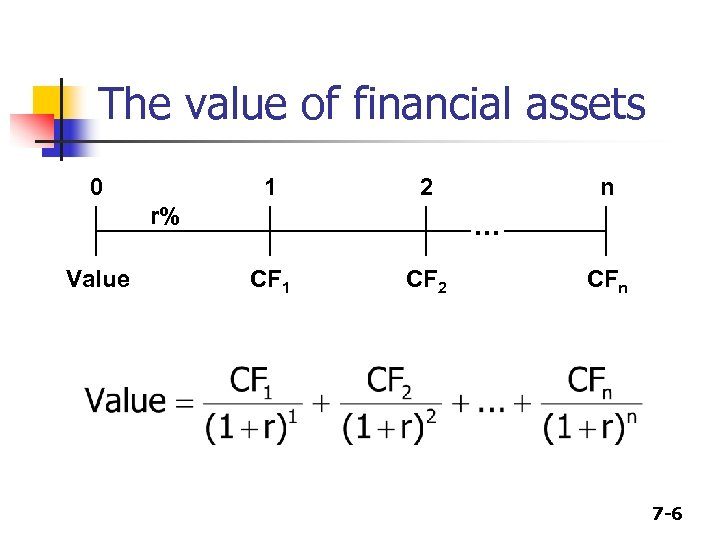The value of financial assets 0 1 2 r% Value n . . . CF 1 CF 2 CFn 7 -6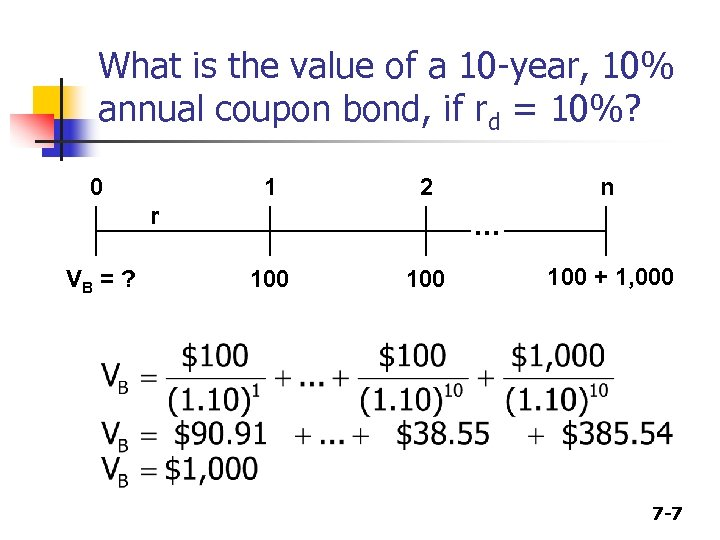What is the value of a 10 -year, 10% annual coupon bond, if rd = 10%? 0 1 2 r VB = ? n . . . 100 100 + 1, 000 7 -7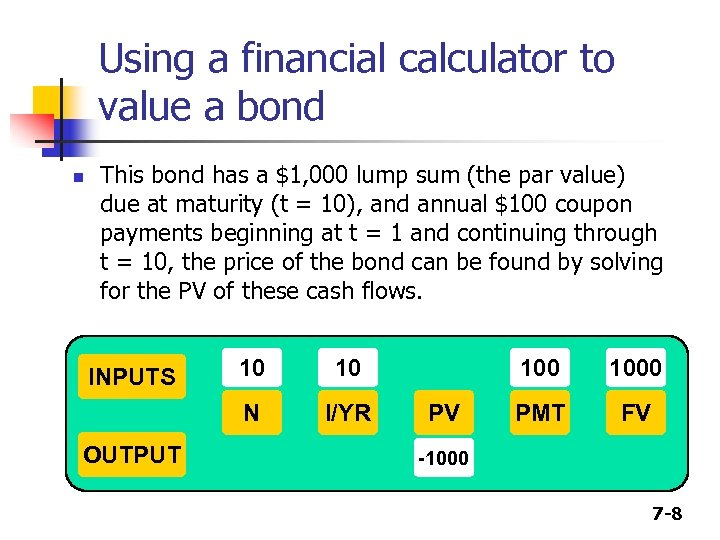Using a financial calculator to value a bond n This bond has a \$1, 000 lump sum (the par value) due at maturity (t = 10), and annual \$100 coupon payments beginning at t = 1 and continuing through t = 10, the price of the bond can be found by solving for the PV of these cash flows. OUTPUT 10 10 N INPUTS I/YR 100 PV 1000 PMT FV -1000 7 -8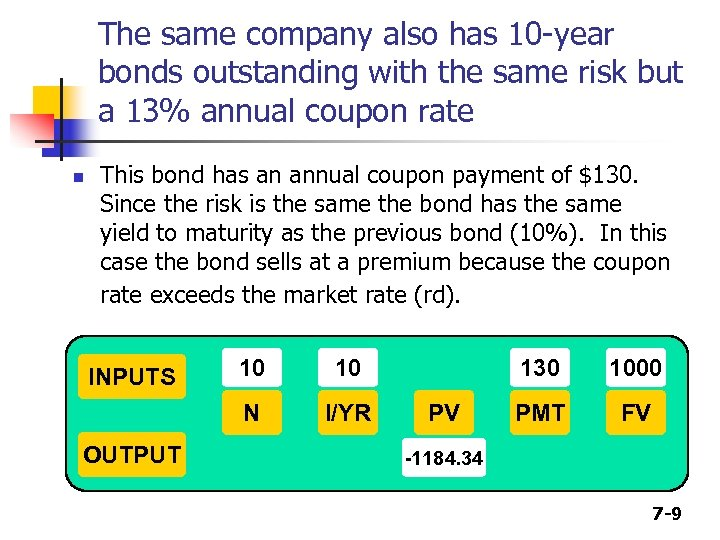The same company also has 10 -year bonds outstanding with the same risk but a 13% annual coupon rate n This bond has an annual coupon payment of \$130. Since the risk is the same the bond has the same yield to maturity as the previous bond (10%). In this case the bond sells at a premium because the coupon rate exceeds the market rate (rd). OUTPUT 10 10 N INPUTS I/YR 130 PV 1000 PMT FV -1184. 34 7 -9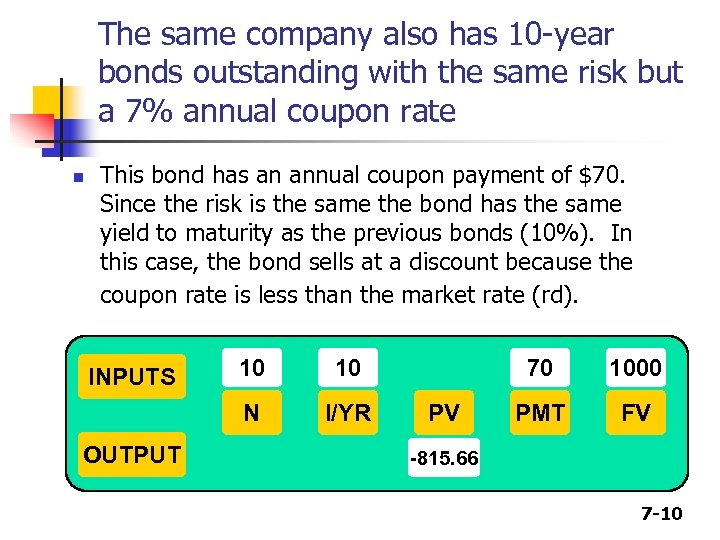The same company also has 10 -year bonds outstanding with the same risk but a 7% annual coupon rate n This bond has an annual coupon payment of \$70. Since the risk is the same the bond has the same yield to maturity as the previous bonds (10%). In this case, the bond sells at a discount because the coupon rate is less than the market rate (rd). OUTPUT 10 10 N INPUTS I/YR 70 PV 1000 PMT FV -815. 66 7 -10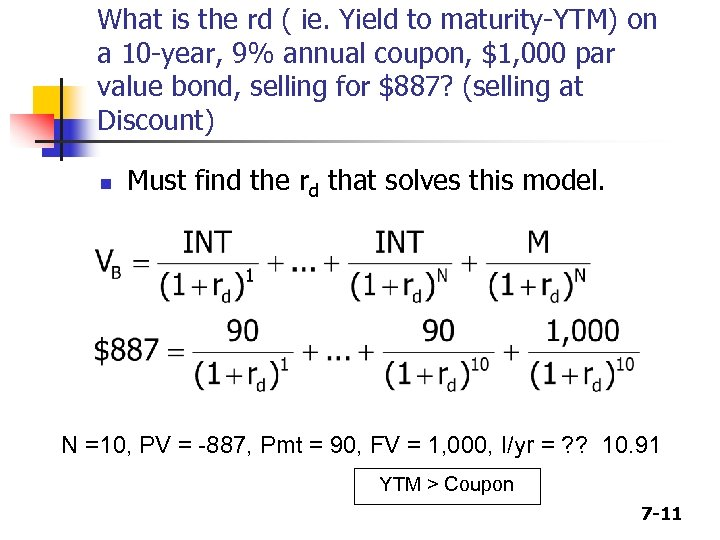What is the rd ( ie. Yield to maturity-YTM) on a 10 -year, 9% annual coupon, \$1, 000 par value bond, selling for \$887? (selling at Discount) n Must find the rd that solves this model. N =10, PV = -887, Pmt = 90, FV = 1, 000, I/yr = ? ? 10. 91 YTM > Coupon 7 -11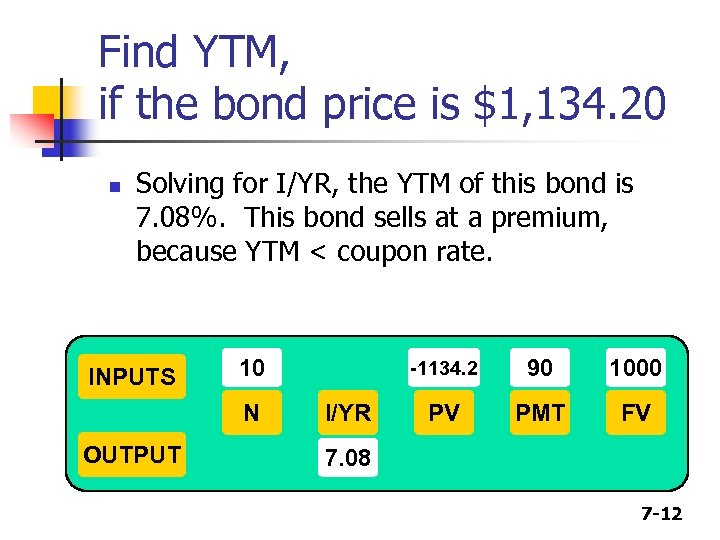Find YTM, if the bond price is \$1, 134. 20 n Solving for I/YR, the YTM of this bond is 7. 08%. This bond sells at a premium, because YTM < coupon rate. INPUTS 10 N OUTPUT -1134. 2 I/YR 90 1000 PV PMT FV 7. 08 7 -12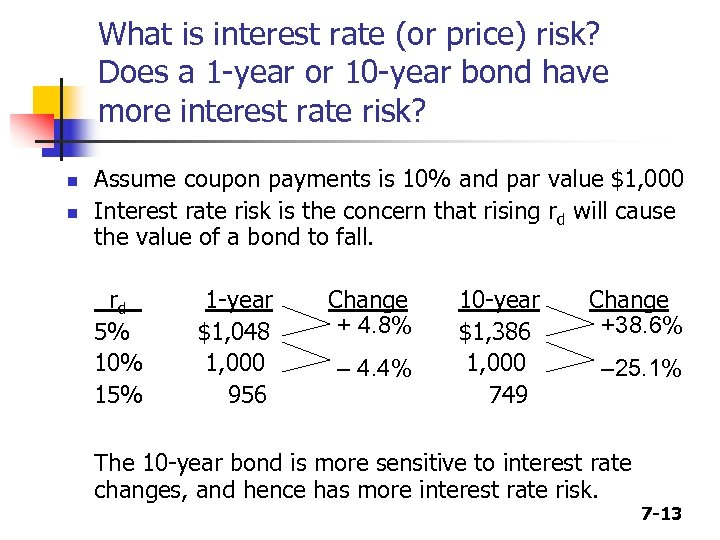What is interest rate (or price) risk? Does a 1 -year or 10 -year bond have more interest rate risk? n n Assume coupon payments is 10% and par value \$1, 000 Interest rate risk is the concern that rising rd will cause the value of a bond to fall. rd 5% 10% 15% 1 -year \$1, 048 1, 000 956 Change + 4. 8% – 4. 4% 10 -year \$1, 386 1, 000 749 Change +38. 6% – 25. 1% The 10 -year bond is more sensitive to interest rate changes, and hence has more interest rate risk. 7 -13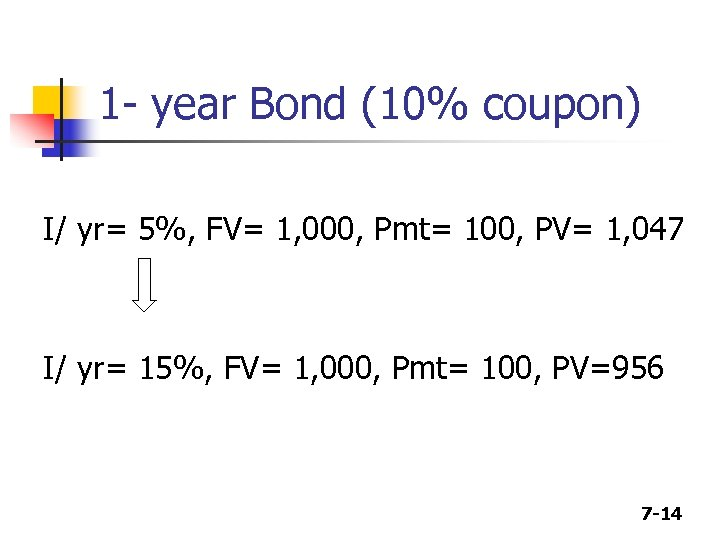1 - year Bond (10% coupon) I/ yr= 5%, FV= 1, 000, Pmt= 100, PV= 1, 047 I/ yr= 15%, FV= 1, 000, Pmt= 100, PV=956 7 -14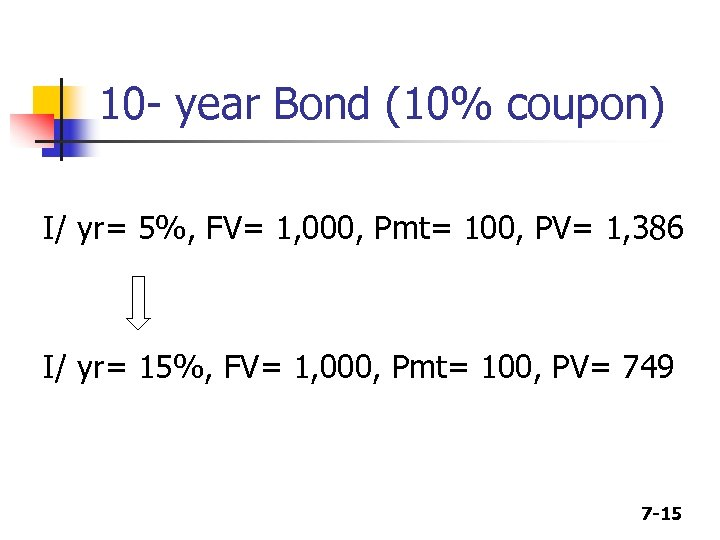10 - year Bond (10% coupon) I/ yr= 5%, FV= 1, 000, Pmt= 100, PV= 1, 386 I/ yr= 15%, FV= 1, 000, Pmt= 100, PV= 749 7 -15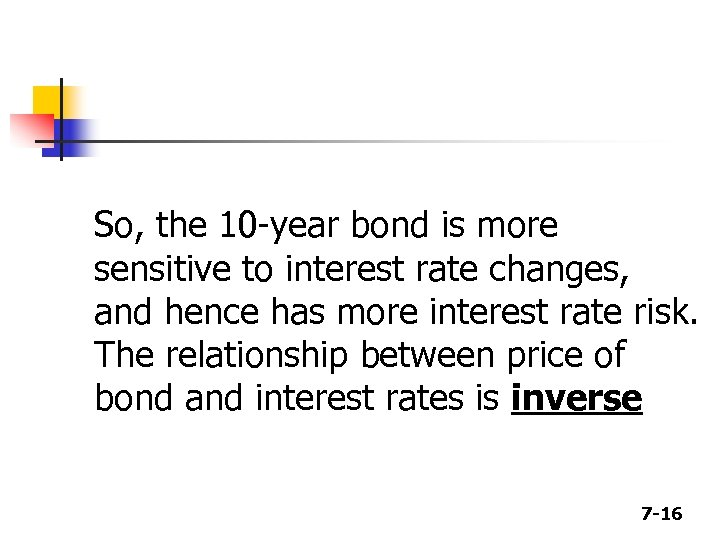So, the 10 -year bond is more sensitive to interest rate changes, and hence has more interest rate risk. The relationship between price of bond and interest rates is inverse 7 -16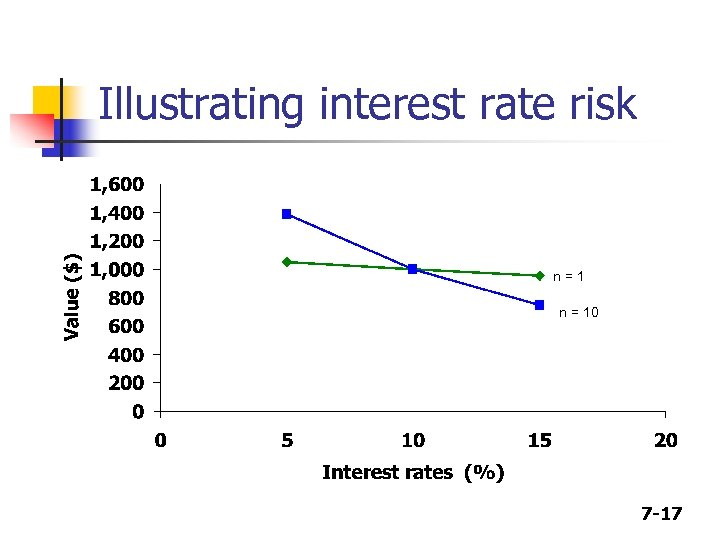Illustrating interest rate risk n=1 n = 10 7 -17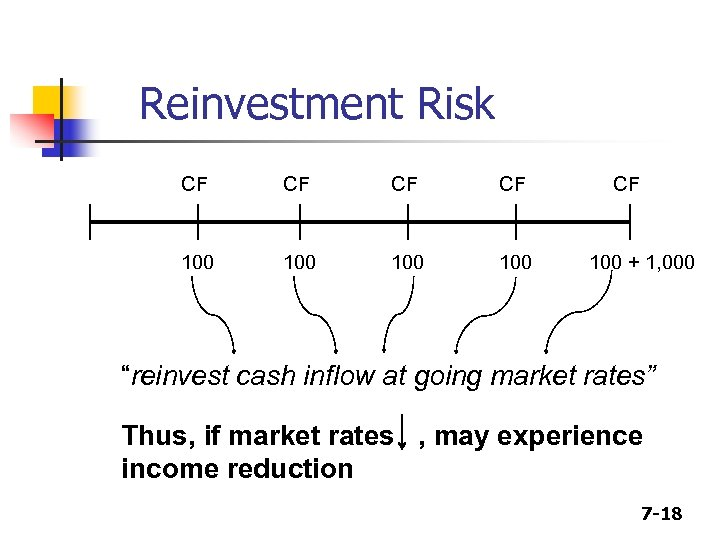Reinvestment Risk CF CF 100 100 CF 100 + 1, 000 “reinvest cash inflow at going market rates” Thus, if market rates , may experience income reduction 7 -18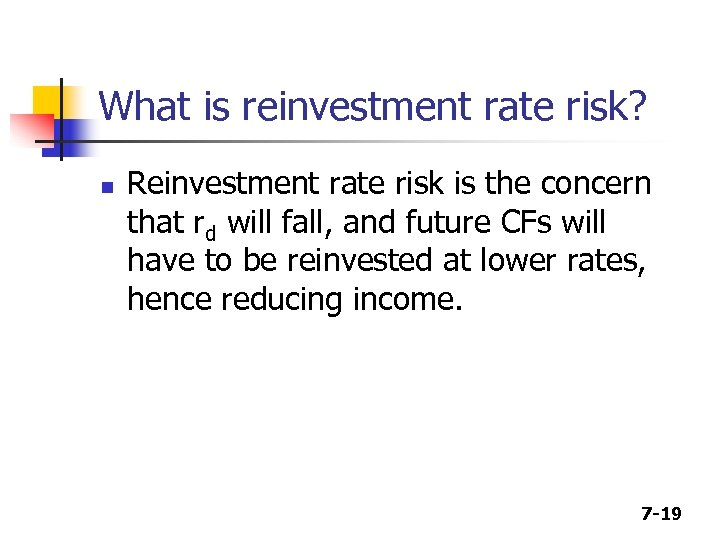What is reinvestment rate risk? n Reinvestment rate risk is the concern that rd will fall, and future CFs will have to be reinvested at lower rates, hence reducing income. 7 -19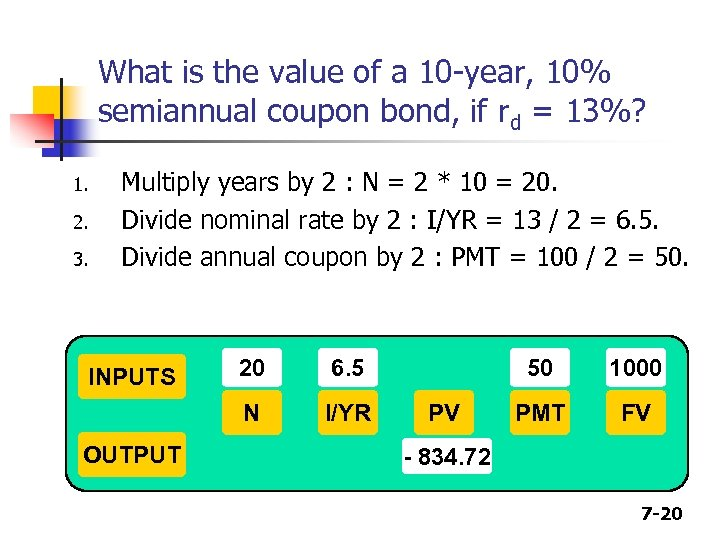What is the value of a 10 -year, 10% semiannual coupon bond, if rd = 13%? 1. 2. 3. Multiply years by 2 : N = 2 * 10 = 20. Divide nominal rate by 2 : I/YR = 13 / 2 = 6. 5. Divide annual coupon by 2 : PMT = 100 / 2 = 50. OUTPUT 20 6. 5 N INPUTS I/YR 50 PV 1000 PMT FV - 834. 72 7 -20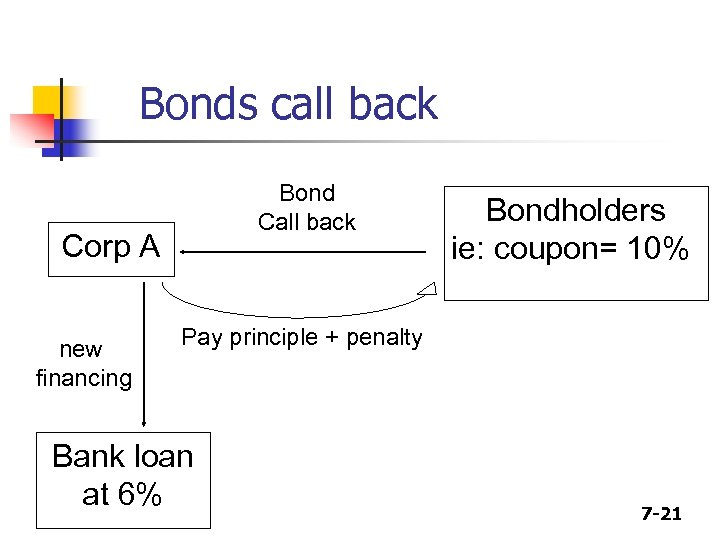Bonds call back Bond Call back Corp A new financing Bondholders ie: coupon= 10% Pay principle + penalty Bank loan at 6% 7 -21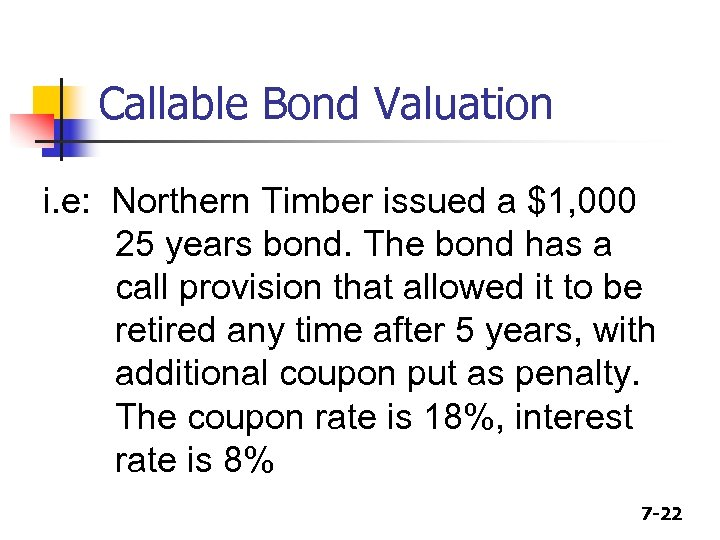Callable Bond Valuation i. e: Northern Timber issued a \$1, 000 25 years bond. The bond has a call provision that allowed it to be retired any time after 5 years, with additional coupon put as penalty. The coupon rate is 18%, interest rate is 8% 7 -22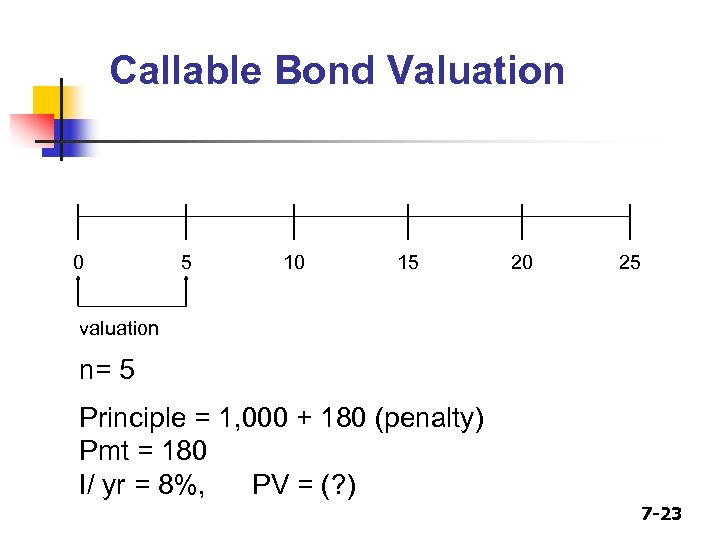Callable Bond Valuation 0 5 10 15 20 25 valuation n= 5 Principle = 1, 000 + 180 (penalty) Pmt = 180 I/ yr = 8%, PV = (? ) 7 -23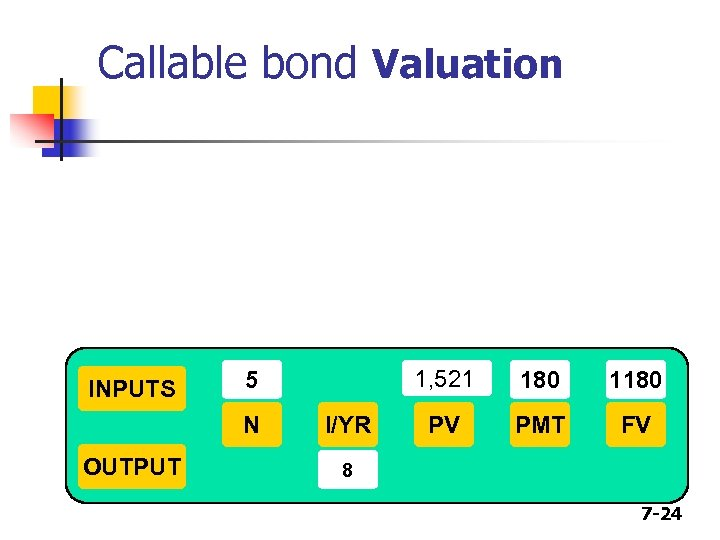Callable bond Valuation INPUTS N OUTPUT 1, 521 5 I/YR 180 1180 PV PMT FV 8 7 -24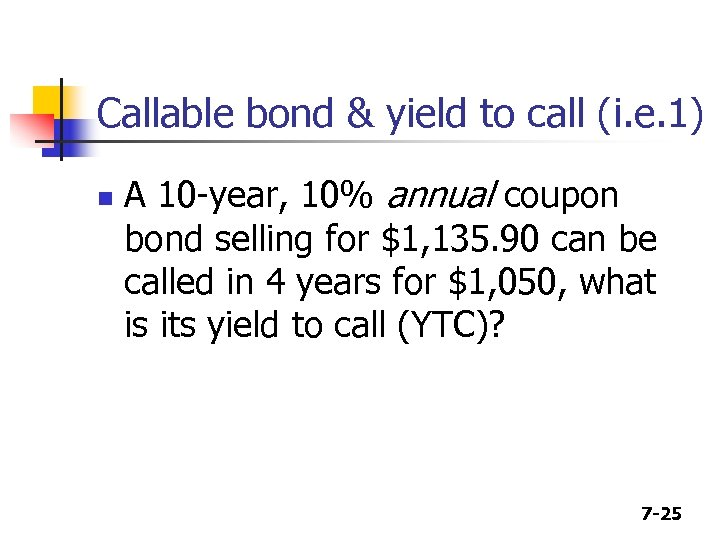Callable bond & yield to call (i. e. 1) n A 10 -year, 10% annual coupon bond selling for \$1, 135. 90 can be called in 4 years for \$1, 050, what is its yield to call (YTC)? 7 -25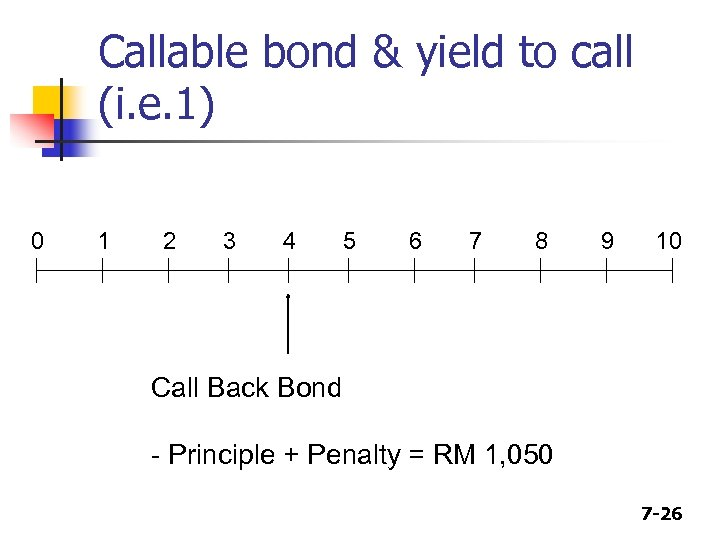Callable bond & yield to call (i. e. 1) 0 1 2 3 4 5 6 7 8 9 10 Call Back Bond - Principle + Penalty = RM 1, 050 7 -26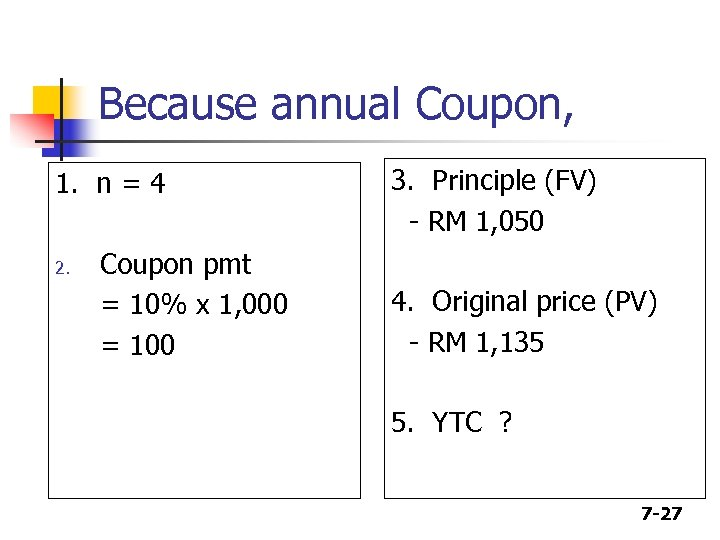Because annual Coupon, 1. n = 4 2. Coupon pmt = 10% x 1, 000 = 100 3. Principle (FV) - RM 1, 050 4. Original price (PV) - RM 1, 135 5. YTC ? 7 -27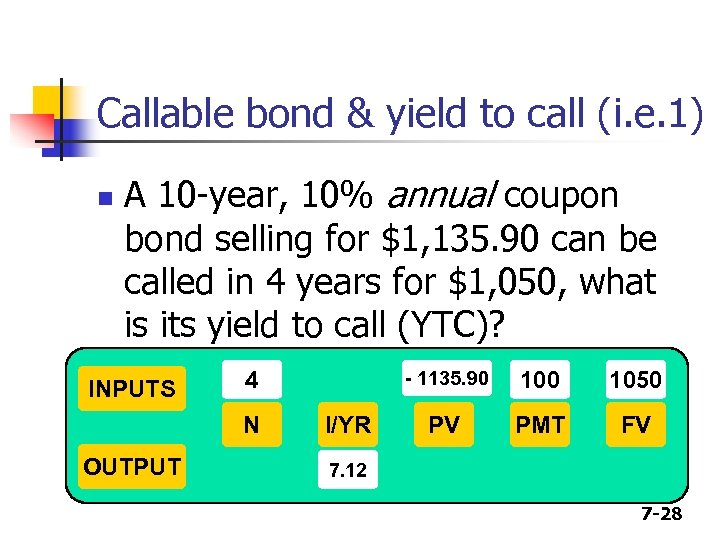Callable bond & yield to call (i. e. 1) n A 10 -year, 10% annual coupon bond selling for \$1, 135. 90 can be called in 4 years for \$1, 050, what is its yield to call (YTC)? INPUTS 4 N OUTPUT - 1135. 90 I/YR 100 1050 PV PMT FV 7. 12 7 -28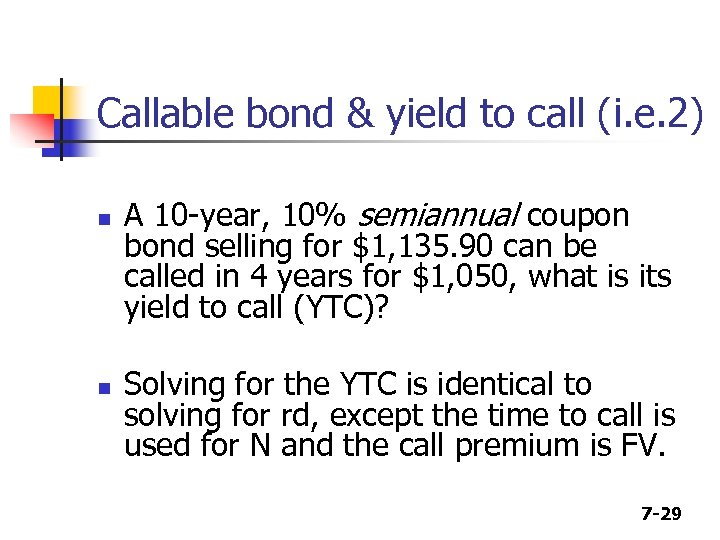Callable bond & yield to call (i. e. 2) n n A 10 -year, 10% semiannual coupon bond selling for \$1, 135. 90 can be called in 4 years for \$1, 050, what is its yield to call (YTC)? Solving for the YTC is identical to solving for rd, except the time to call is used for N and the call premium is FV. 7 -29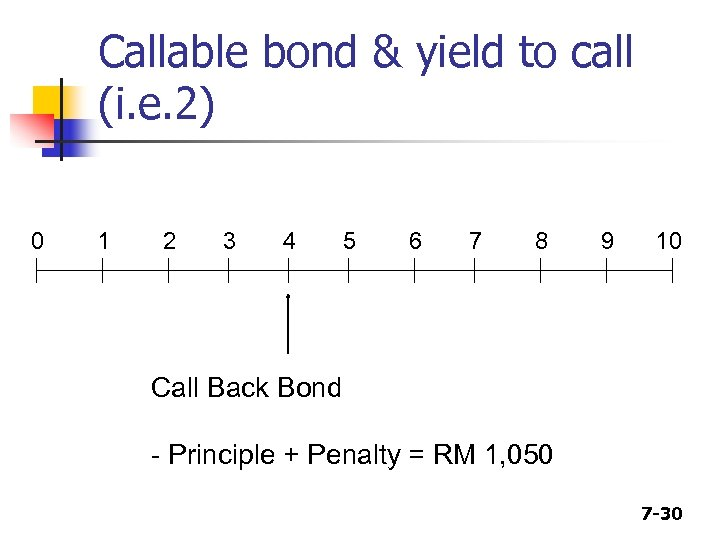Callable bond & yield to call (i. e. 2) 0 1 2 3 4 5 6 7 8 9 10 Call Back Bond - Principle + Penalty = RM 1, 050 7 -30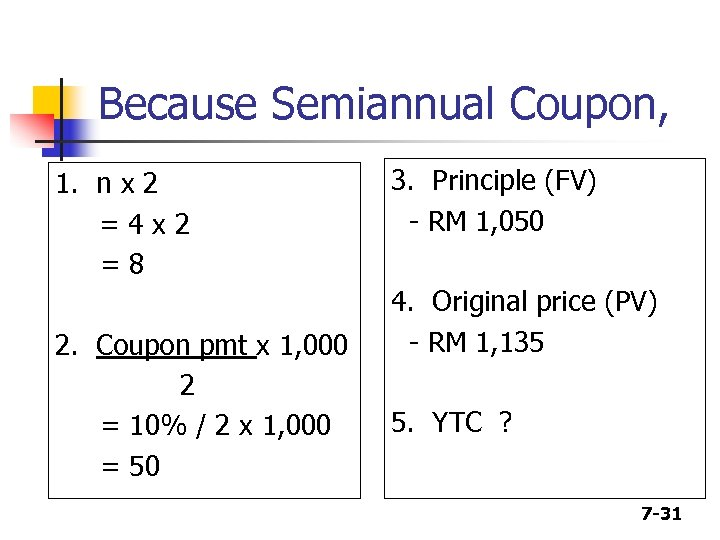Because Semiannual Coupon, 1. n x 2 =4 x 2 =8 2. Coupon pmt x 1, 000 2 = 10% / 2 x 1, 000 = 50 3. Principle (FV) - RM 1, 050 4. Original price (PV) - RM 1, 135 5. YTC ? 7 -31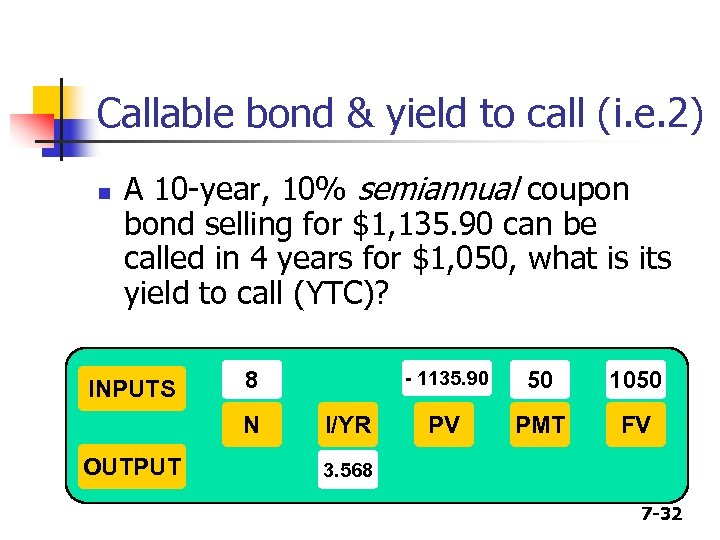Callable bond & yield to call (i. e. 2) n A 10 -year, 10% semiannual coupon bond selling for \$1, 135. 90 can be called in 4 years for \$1, 050, what is its yield to call (YTC)? INPUTS 8 N OUTPUT - 1135. 90 I/YR 50 1050 PV PMT FV 3. 568 7 -32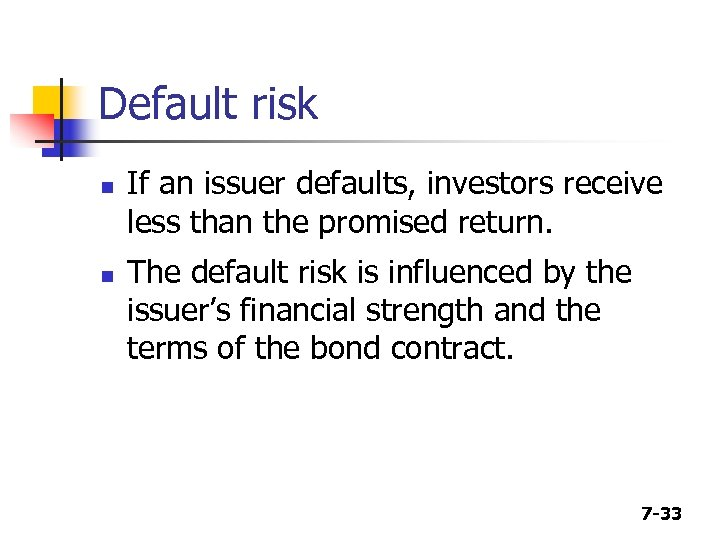Default risk n n If an issuer defaults, investors receive less than the promised return. The default risk is influenced by the issuer’s financial strength and the terms of the bond contract. 7 -33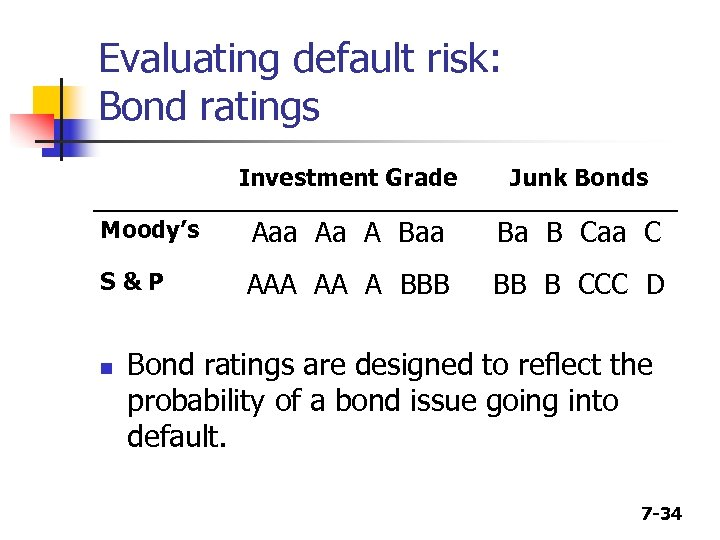Evaluating default risk: Bond ratings Investment Grade Junk Bonds Moody’s Aaa Aa A Baa Ba B Caa C S&P AAA AA A BBB BB B CCC D n Bond ratings are designed to reflect the probability of a bond issue going into default. 7 -34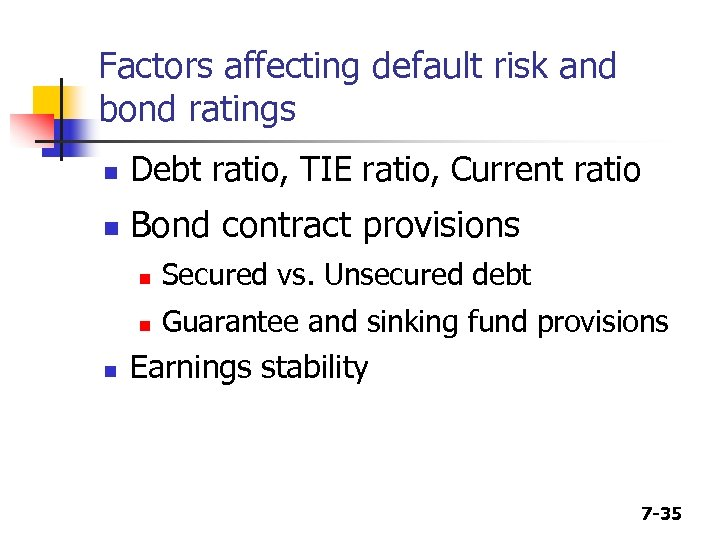Factors affecting default risk and bond ratings n Debt ratio, TIE ratio, Current ratio n Bond contract provisions n n n Secured vs. Unsecured debt Guarantee and sinking fund provisions Earnings stability 7 -35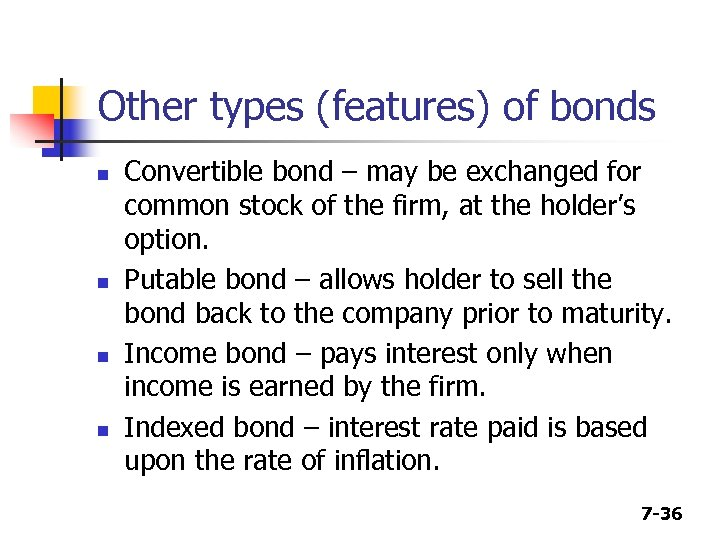Other types (features) of bonds n n Convertible bond – may be exchanged for common stock of the firm, at the holder’s option. Putable bond – allows holder to sell the bond back to the company prior to maturity. Income bond – pays interest only when income is earned by the firm. Indexed bond – interest rate paid is based upon the rate of inflation. 7 -36